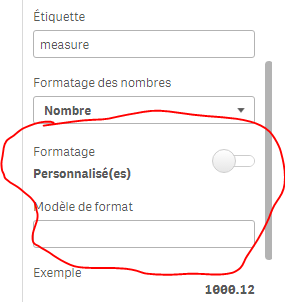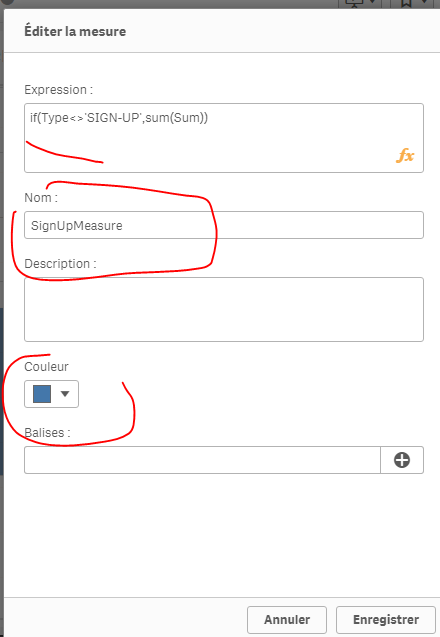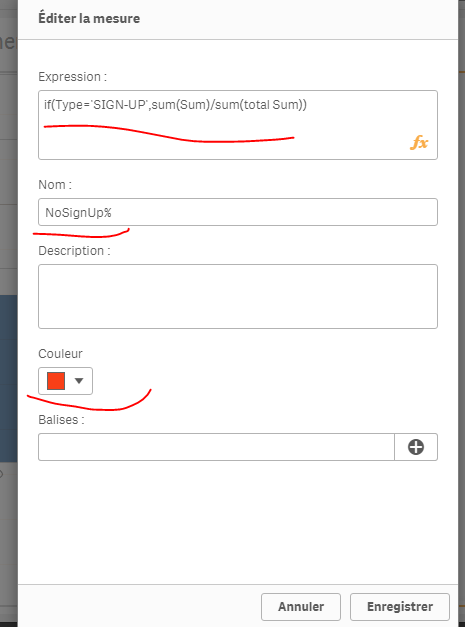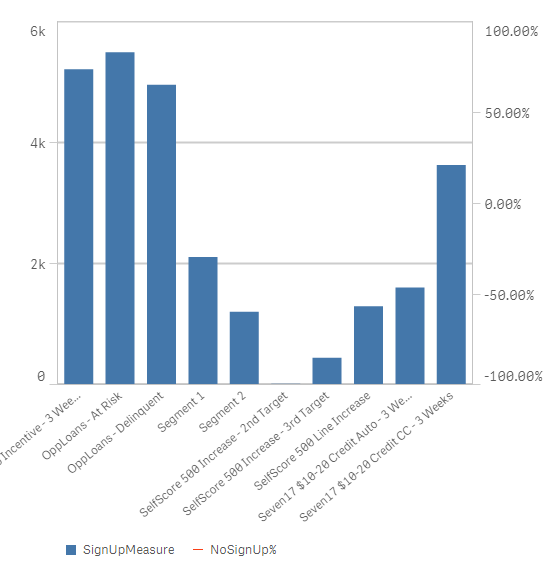# New to Qlik Sense

Discussion board where members can get started with Qlik Sense.

Announcements
BARC’s The BI Survey 19 makes it official. BI users love Qlik. GET REPORT
HighlightedNot applicable

## How to change the values in measure as percentage?

Hello All,From the above graph

In delivered emails i have values but in open rate i need those values should be in percentage type as well as in signup rate too.

1 Solution

Accepted SolutionsPartner

## Re: How to change the values in measure as percentage?

I found out 2 "solutions"; I don't know if it's appealing enough though..
1st one:

in the same graphic, having some dimensions having sum(Sum) and others as % (but you'll have to know what's the % because I couldn't show the % sign):

Measure :

if(Type='SIGN-UP',num(sum(Sum),'##0,00'), num((sum(Sum)/sum(total Sum))*100,'##0,00%'))result:2) you'll have to use a combined chart and thus, you can't have 2 dimensions in your chart but only ONLY hiearchic dimension :and create 2 master measures:Now, in your chart, use these dimension and measures :

result:I click on open and confirm : I'll have sum(Sum) by segments of type open:see the attached app

You can also have the information of the % for juste the Type OPEN for example:

Type to =Type& ' '&aggr( if(Type='OPEN',num((sum(Sum)/sum(total Sum))*100,'##0,00%')),Type)

Segment_Name to =Segment_Name& ' '&aggr( if(Type='OPEN',num((sum(Sum)/sum(total Sum))*100,'##0,00%')),Segment_Name,Type)

result :13 Replies
Valued Contributor III

## Re: How to change the values in measure as percentage?

I think this is not possible , but if you hover on the bars you will see the Value and SharePartner

## Re: How to change the values in measure as percentage?

Unfortunately, it's not possible to control what's being seen in the pop up in Qlik Sense; the only way to do it is to color by expression, and the expression would be the %; in that case, when you hover on it, you'll see the percentage.

mto‌, in qlikView, we have the possibility to control what's being seen when we hover over a dimension; we could add a measure, make it invisible and it will only appear when we hover over an object.
Is such a possiblity or another direct way (like in tableau) is thought about in the next relase?

Thanks,Not applicable

## Re: How to change the values in measure as percentage?

Hi Goutham

If you looking to convert in % here you are:

1. num((YourExpression), '#,##0%')

2. Number formatting should be Number

3. Formatting should be simple and select 12% from drop downNot applicable

## Re: How to change the values in measure as percentage?

But for Open Rate it should be in percentage, and for delivered emails it must be in valuesPartner

## Re: How to change the values in measure as percentage?

and for the others?
Can you share your app with just this chart on it? I'll try sthingNot applicable

## Re: How to change the values in measure as percentage?

Okay bro,,Not applicable

## Re: How to change the values in measure as percentage?

omarbensalem‌ !

I want Delivered Emails as same(Values) and Open Rate in percentagePartner

## Re: How to change the values in measure as percentage?

I found out 2 "solutions"; I don't know if it's appealing enough though..
1st one:

in the same graphic, having some dimensions having sum(Sum) and others as % (but you'll have to know what's the % because I couldn't show the % sign):

Measure :

if(Type='SIGN-UP',num(sum(Sum),'##0,00'), num((sum(Sum)/sum(total Sum))*100,'##0,00%'))result:2) you'll have to use a combined chart and thus, you can't have 2 dimensions in your chart but only ONLY hiearchic dimension :and create 2 master measures:Now, in your chart, use these dimension and measures :

result:I click on open and confirm : I'll have sum(Sum) by segments of type open:see the attached app

You can also have the information of the % for juste the Type OPEN for example:

Type to =Type& ' '&aggr( if(Type='OPEN',num((sum(Sum)/sum(total Sum))*100,'##0,00%')),Type)

Segment_Name to =Segment_Name& ' '&aggr( if(Type='OPEN',num((sum(Sum)/sum(total Sum))*100,'##0,00%')),Segment_Name,Type)

result :Not applicable

## Re: How to change the values in measure as percentage?

First option i can implement, but i need one more clarification,

I need both OPEN and SIGN-UP should be in percentage

if(Type='OPEN',num((sum(Sum)/sum(total Sum))*100,'##0,00%'), num(sum(Sum),'##0,00'))

Above formula works only for OPEN, but in the same formula can i insert SIGN-Up.

Is it correct=if(Type='OPEN' AND 'SIGN-UP',num((sum(Sum)/sum(total Sum))*100,'##0,00%'), num(sum(Sum),'##0,00'))